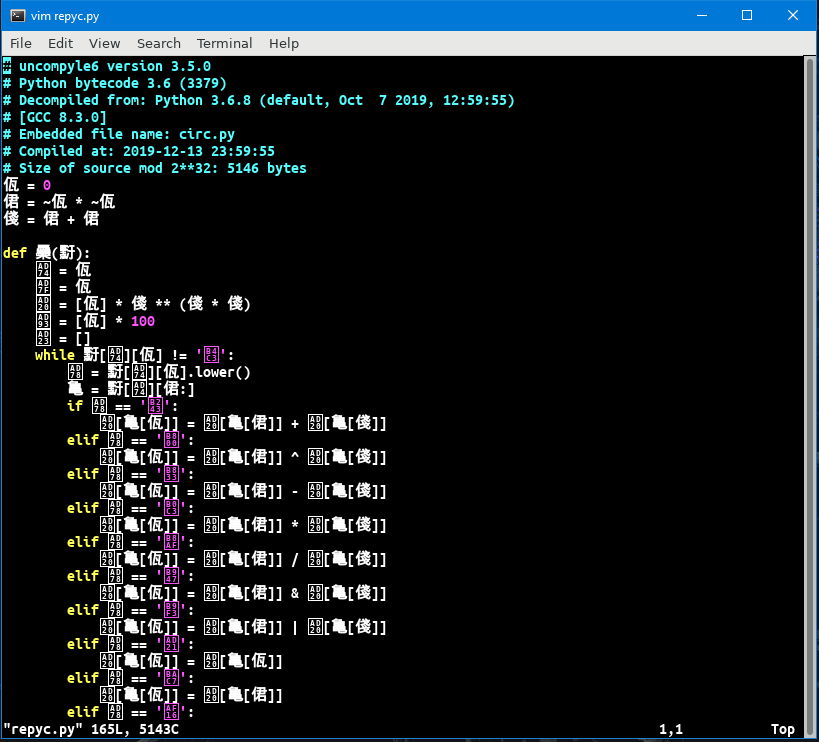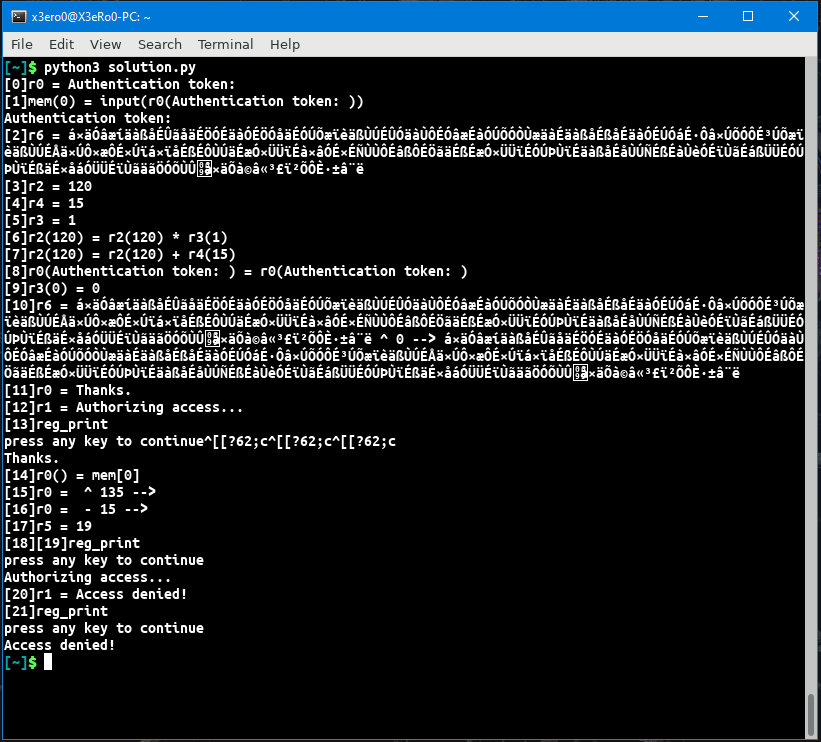### Challenge: REPYC [REV] - 147 Points

Challenge Description:

• woo thi chal sooo repyc!
• File: 3nohtyp.pyc
• Difficulty estimate: Easy

We are given a pyc file and running file on this pyc reveals that its a python 3.6 compiled python bytecode.

``````1[~]\$ file 3nohtyp.pyc
23nohtyp.pyc: python 3.6 byte-compiled``````

so i went with the usuall approach to a python pyc challenge, where the first part is to decompile the bytecode. i used uncompyle6 `[~]\$ uncompyle6 3nohtyp.pyc > repyc.py` and the output was very weird just look at this fileI never thought that reversing a python source file would be so difficult. At first i thought maybe there is python obfuscator which obfuscates variable names with unicode Chinese looking charecters and there are a lot of other obfuscators which uses other techniques similar to this one but unfortunately i didn’t find any obfuscator which does this kind of obfuscation, i thought if i found the obfuscator maybe an unobfuscator also exists so that will make my work easier but i was wrong.

Some good obfuscators which i found are:

So the next thing i did was i started replacing each chinese charecter in the file with simple names like i translated first 3 lines like this

``````1佤 = 0
2侰 = ~佤 * ~佤
3俴 = 侰 + 侰
``````

translated to

``````1A = 0
2B = ~A * ~A
3C = B + B
``````

and finally

``````1A = 0
2B = 1
3C = 2
``````

And later in the code instead of just using 1, 2 and 3 they use A, B and C so i replaced all the A, B and Cs with their respective values

then comes this function

``````1def 䯂(䵦):
``````

At this point i already guessed it was a VM so i named this function VM(byte)

next we see some more declarations which turns out to be registers and memory and stack and stuff for example

`괠 = [A] * C ** (C * C)` translates to `reg =  * 16` and `궓 = [A] * 100` translates to `mem =  * 100` and after reading the rest of the code turns out `괣 = []` was the stack

so this part looks like this now

`````` 1A = 0
2B = 1
3C = 2
4
5def VM(byte):
6    var1 = 0
7    var2 = 0
8    reg  =  * 16 # array of registers
9    mem =  * 100 # contains our input
10    stack = []
``````

then there is a while loop

``1 while 䵦[굴][A] != '듃':``
which translated to
``1 byte[var1] != '듃' ``
so it was clear that ‘듃’ is the hlt instruction as soon as it encounters ‘듃’ the VM will stop execution

and finally

``````1굸 = 䵦[굴][A].lower()
2亀 = 䵦[굴][B:]
``````

translated to

``````1opcode = byte[var1].lower()
2_arg_ = byte[var1][1:]
``````

and at this point it was time to analyse all the opcodes, so i used my big brain and only analysed the ones which were actually executed. when you see the call to the VM() function the arguments passed is a list of instructions for sat down and started to do find and replace on all the instructions. but then i noticed that the instructions in the bottom were not correctly indented so i fixed that and i ended up with thisafter that i just did a lot of find and replace and i finally fixed the whole python file and i ended up with this VM Bytecode

`````` 1VM([
2 ['mov', 0, 'Authentication token: '],
3 ['mem_mov', 0, 0], # mem(r1) = r2
5 ['mov', 2, 120],
6 ['mov', 4, 15],
7 ['mov', 3, 1],
8 ['multiply', 2, 2, 3], # r2 = r2 * r3
9 ['add', 2, 2, 4], # r2 = r2 + r4
10 ['nop', 0, 2], # maybe nop
11 ['zero_reg', 3], # sets r3 = 0
12 ['xor_string', 6, 3],
13 ['mov', 0, 'Thanks.'],
14 ['mov', 1, 'Authorizing access...'],
15 ['print_reg', 0], # prints r0
16 ['reg2mem', 0, 0], # set r[arg0] = mem[arg1]
17 ['xor_string', 0, 2],
18 ['subtract_string', 0, 4],
19 ['mov', 5, 19],
20 ['strcmp', 0, 6, 5], # check strings ; if not equal r = 1 ; push r[arg]
21 ['print_reg', 1],
23 ['print_reg', 1],
24 ['hlt']])
``````

clearly it loads this huge string into r6 and then xors it with 135 and then subtract 15 from each charecter and then compares with our input so i quicly wrote a decrypt function

`````` 1def find_flag():
2
4    temp = ''
5    for i in range(len(b)):
6        temp += chr(ord(b[i]) + 15)
7
8    a = temp
9    temp = ""
10    for i in range(len(a)):
11        temp += chr(ord(a[i]) ^ 135)
12
13    print(temp)
``````

and just after that i got the flag

``````1[~]\$ python3 solution.py